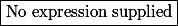# Matematické Fórum

Nevíte-li si rady s jakýmkoliv matematickým problémem, toto místo je pro vás jako dělané.

Nástěnka
🔒 23. 3. 2019 Přešli jsme na HTTPS. Prosíme o kontrolu funkčnosti fóra.
!! 17.06.2018 (Jel.) Khanova škola zve nadšence ke spolupráci na překladech návodů pro učitele a rodiče.
! 04.11.2016 (Jel.) Čtete, prosím, před vložení dotazu, děkuji!
17.01.2016 (Jel.) Rok 2016 s novými a novějšími krystaly od kolegy Pavla!
17.01.2016 (Jel.) Nabídka knih z oborů matematiky, fyziky, chemie
23.10.2013 (Jel.) Zkuste před zadáním dotazu použít některý z online-nástrojů, konzultovat použití můžete v sekci CAS.

Nejste přihlášen(a). Přihlásit

## #1 28. 04. 2018 20:41 — Editoval stuart clark (01. 05. 2018 10:57)

stuart clarkPříspěvky: 935
Reputace:

### roots of function

If$f(x)$ is twice differentiable function on$[a,e]$ and for [Image_Link]//www.matematika.cz/cgi-bin/mathtex.cgi?\dpi{140}\gammacorrection{1}\parstyle\begin{align*}\usepackage[czech]{babel} a

Then minimum number of roots of the equation$f(x)f''(x)+(f'(x))^2=0$ is

Offline

## #2 28. 04. 2018 21:09 — Editoval laszky (02. 05. 2018 01:05)

laszky
Příspěvky: 1372
Škola: MFF UK, FJFI CVUT
Reputace:   105

Hi

Offline

## #3 01. 05. 2018 10:56

stuart clarkPříspěvky: 935
Reputace:

### Re: roots of function

↑ laszky: Thanks .

Offline

## #4 01. 05. 2018 10:58

stuart clarkPříspěvky: 935
Reputace:

### Re: roots of function

Please explain

If$f(x)$ is twice differentiable function on$[a,e]$ and for [Image_Link]//www.matematika.cz/cgi-bin/mathtex.cgi?\dpi{140}\gammacorrection{1}\parstyle\begin{align*}\usepackage[czech]{babel} a

Then minimum number of roots of the equation$f(x)f''(x)+(f'(x))^2=0$ is

Offline

## #5 02. 05. 2018 01:30 — Editoval laszky (02. 05. 2018 13:21)

laszky
Příspěvky: 1372
Škola: MFF UK, FJFI CVUT
Reputace:   105

### Re: roots of function

↑ stuart clark:

Since$\frac{1}{2}f^2(x)$ is non-negative and has zeroes at$a$,$e$ and somewhere between$b$,$c$ and$c$,$d$, there exist at least 6 roots of$g(x)=\frac{1}{2}f^2(x)-\frac{1}{2}$ in$[a,e]$. Consequently, as in the previous case, there exist at least 4 roots of$g''(x)=\left(\frac{1}{2}f^2(x)-\frac{1}{2}\right)''= f(x)f''(x)+(f'(x))^2=0$ in$[a,e]$.

Offline

## #6 03. 05. 2018 07:10 — Editoval stuart clark (03. 05. 2018 09:12)

stuart clarkPříspěvky: 935
Reputace:

### Re: roots of function

Thanks ↑ laszky: But answer Given as$6$.

i have one doubt How can we assume function$g(x)=\frac{(f(x))^2}{2}-1$ in first  question. (We can assume other function also like$g(x)=\frac{(f(x))^2}{2}-2$ type

Similarly How can we assume function$g(x)=\frac{(f(x))^2}{2}-\frac{1}{2}.$ for second question. We can also assume other function.

please clearfy me , thanks in advanced.

Offline

## #7 03. 05. 2018 10:43

laszky
Příspěvky: 1372
Škola: MFF UK, FJFI CVUT
Reputace:   105

### Re: roots of function

↑ stuart clark:

The crucial words are "at least".  At first you have to notice that$\left(\frac{1}{2}f^2(x)\right)''=f(x)f''(x)+(f'(x))^2$, then you observe that$\left(\frac{1}{2}f^2(x)\right)''=\left(\frac{1}{2}f^2(x)-ax-b\right)''$ for arbitrary$a,b\in\mathbb{R}$ and you choose$a,b$ in such a way that$g(x)=\frac{1}{2}f^2(x)-ax-b$ has maximal possible zeros. If you choose them wrong, you prove only partial result (not the optimal one) - like me in my previous answer. (I forgot that you can substract any linear function and substracted only constant). My approach is: draw a picture of the presumable graph of the function$\frac{1}{2}f^2(x)$ and then try to draw a line which intersects the function$\frac{1}{2}f^2(x)$ in maximal possible points.

The following picture shows my first attempt with 6 zeros of the function g and my second attempt with 7 zeros of the function g (due to the zero derivative of$\frac{1}{2}f^2(x)$ there are two intersections near the point$a$ ). Hence, using this second attempt, we can prove that there exists at least 5 roots of$f(x)f''(x)+(f'(x))^2$. But 6 is still problem for me.

Offline

## #8 05. 05. 2018 04:11

stuart clarkPříspěvky: 935
Reputace:

### Re: roots of function

Thanks ↑ laszky:.

Offline

## Zápatí

Powered by PunBB
© Copyright 2002–2005 Rickard Andersson